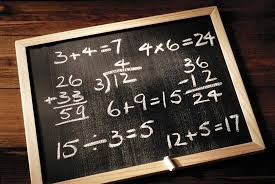# Difference between Algebra and Arithmetic

##### Key Difference:  Arithmetic and Algebra are two branches of Mathematics. Arithmetic, being the most basic of all branches of mathematics, deals with the basic computation of numbers by using operations like addition, multiplication, division and subtraction. On the other hand, Algebra uses numbers and variables for solving problems. It is based on application of generalized rules for problem solving.Arithmetic and Algebra are two different branches of mathematics. Arithmetic, the term itself has been derived from a Greek word meaning number. It is the most basic branch of mathematics. It is all about numbers, and therefore is commonly used by everyone in day to day life. Elementary Arithmetic works around four main operations which are addition, subtraction, division and multiplication. It simply uses numbers for various types of calculations

Higher Arithmetic is also known as number theory. It is concerned with characteristics of integers, rational numbers, irrational numbers and real numbers.On the other hand, Algebra is another branch of mathematics. The word is derived from the Arabic word al-jabr, which is an ancient medical term is meaning the ‘reunion of broken parts’. It can be considered as the next level of mathematics after the foundation of arithmetic. Unlike Arithmetic, it deals with unknown quantities in combination with numbers. One can easily identify an algebraic operation with symbols X, Y, a, b, etc. It is mainly concerned with rules for manipulating arithmetic operation.

It involves powers, algorithm and complex numbers also. Algebra uses products and factoring, quadratic formal and binomial theorems, etc. to reach a solution. Basic algebraic properties are used for evaluation of algebraic equations.

For example, 3+7 = 7+3, this is an arithmetic expression

Whereas, a+b=b+a is an algebraic equation, because it will be valid for a number of situations. Arithmetic might show some regularity, whereas algebra would give expression to define these patterns based on the regularities. Thus, Arithmetic can be considered as the computation of certain numbers, whereas Algebra is about generalization of some conditions which will hold true for all number, or all whole numbers, or whole integers, etc.

Unlike elementary arithmetic, elementary algebra uses letter for problem solving. However, higher arithmetic makes use of letters in the same way as used in the rest of mathematics’ domain.

Comparison between Algebra and Arithmetic:

 Arithmetic Algebra Definition Arithmetic, being the most basic of all branches of mathematics, deals with the basic computation of numbers by using operations like addition, multiplication, division and subtraction. Algebra uses numbers and variables for solving problems. It is based on application of generalized rules for problem solving. Level Generally, associated with elementary school mathematics Generally, associated with high school mathematics Computation Method Computation with specific numbers Introduces generality and abstraction related concepts Main focus Four operations (adding, subtracting, multiplication and division) Algebra uses numbers and variables for solving problems. It is based on application of generalized rules for problem solving Problem solving Based on the information provided in the problem (memorized results for small values of numbers) Based on the standard moves of elementary algebra Relation Number related Variable related

Image Courtesy: kids.britannica.com, nuview.k12.ca.us